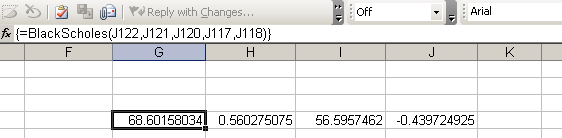Categories

## The CME Globex Electronic Futures Trading System

The story of the Reuters Globex futures trading system developed for the Chicago Mercantile Exchange is a classic ETS (Electronic Trading System) case study. Originally devised in 1987 and launched in 1992, the product was plagued with technical difficulties and defects, and the general reluctance of the traditionally pit-based futures trading community to accept its merits.

On its first day of business in June 1992, 2,063 futures contracts traded on CME Globex. Today, an average of more than one million contracts a day are traded on CME Globex, accounting for over 50% of total CME volume.

The orginal product was beset with issues, and there were still serious doubts as to whether an ETS could ever replace the open outcry system:

However, the success of Globex (although still not without its issues – see http://www.finextra.com/fullstory.asp?id=13768) is a strong validation of the potential efficiency gains that can be realised on an ETS platform.

Categories

## Excel Black-Scholes Function

[UPDATE: After reading this post, see here for an updated version of the spreadsheet].

The Black-Scholes option valuation formula for an option paying a continuous dividend yield is the following:WhereandAttached is a simple Excel function that calculates the Black-Scholes option value for a specific set of input parameters. Currently, it just calculates the call value – if you use it as an array function, it will return a 4-element array with call value, call delta, put value, put delta, respectively. You could extend it pretty easily to calculate the rest of the Greeks.

Here is the code for the function (To create an Excel function, press ALT-F11 in your workbook and select Insert>Module. Note that the dividend yield parameter is optional.

Function BlackScholes(SpotPrice As Double, ExercisePrice As Double,
TimeToMaturity As Double, RiskFreeRate As Double, sigma As Double,
Optional DividendYield As Double) As Double()

Dim d1 As Double
Dim d2 As Double
Dim Nd1 As Double
Dim Nd2 As Double
Dim ResultArray() As Double

ReDim ResultArray(4) As Double

If (IsMissing(DividendYield)) Then
d1 = WorksheetFunction.Ln(SpotPrice / ExercisePrice) +
((RiskFreeRate + (0.5 * (sigma ^ 2))) * TimeToMaturity)
Else
d1 = WorksheetFunction.Ln(SpotPrice / ExercisePrice) +
((RiskFreeRate - DividendYield + (0.5 * (sigma ^ 2))) * TimeToMaturity)
End If

d1 = d1 / (sigma * (TimeToMaturity ^ (1 / 2)))
d2 = d1 - (sigma * (TimeToMaturity ^ (1 / 2)))
Nd1 = WorksheetFunction.NormSDist(d1)
Nd2 = WorksheetFunction.NormSDist(d2)

'Call Value
If (IsMissing(DividendYield)) Then
ResultArray(0) = (SpotPrice * Nd1)
- (ExercisePrice * Exp(-RiskFreeRate * TimeToMaturity) * Nd2)
Else
ResultArray(0) = Exp(-DividendYield * TimeToMaturity) * (SpotPrice * Nd1)
- (ExercisePrice * Exp(-RiskFreeRate * TimeToMaturity) * Nd2)
End If

'Call Delta
ResultArray(1) = Nd1

'Put Value
If (IsMissing(DividendYield)) Then
ResultArray(2) = Exp(-RiskFreeRate * TimeToMaturity)
* ExercisePrice * (1 - Nd2) - SpotPrice * (1 - Nd1)
Else
ResultArray(2) = Exp(-RiskFreeRate * TimeToMaturity) * ExercisePrice
* WorksheetFunction.NormSDist(-d2) - Exp(-DividendYield * TimeToMaturity)
* SpotPrice * WorksheetFunction.NormSDist(-d1)
End If

'Put delta
ResultArray(3) = -WorksheetFunction.NormSDist(-d1)

BlackScholes = ResultArray
End Function


Save this, and set up the input parameters in Excel. Select a range of 4 cells, and then click the f(x) function selection button. Choose from the list of User Defined functions, and then select BlackScholes. Excel will prompt you for the input parameters:When you are finished inputing the parameters, press CTRL+SHIFT+ENTER to execute the function. Excel will populate the four cells with the calculated option values:A sample workbook is attached:BlackScholes.xls